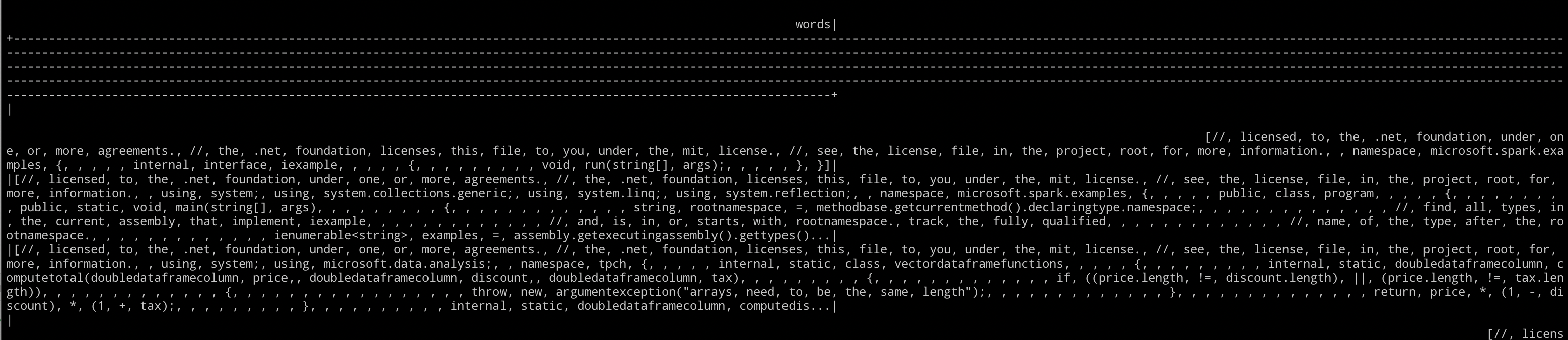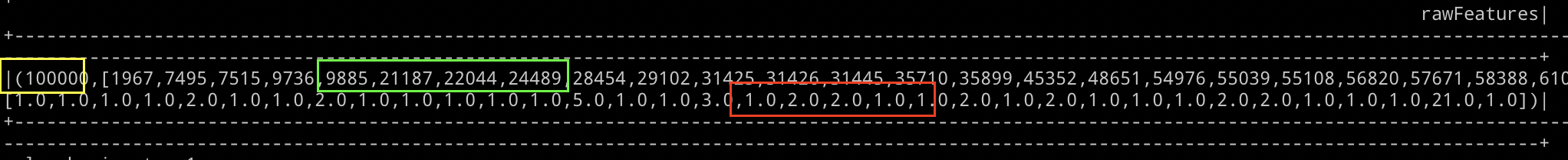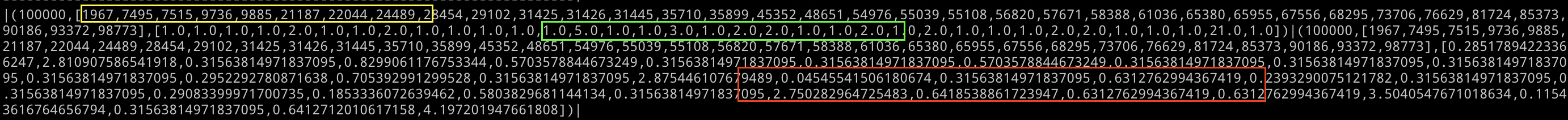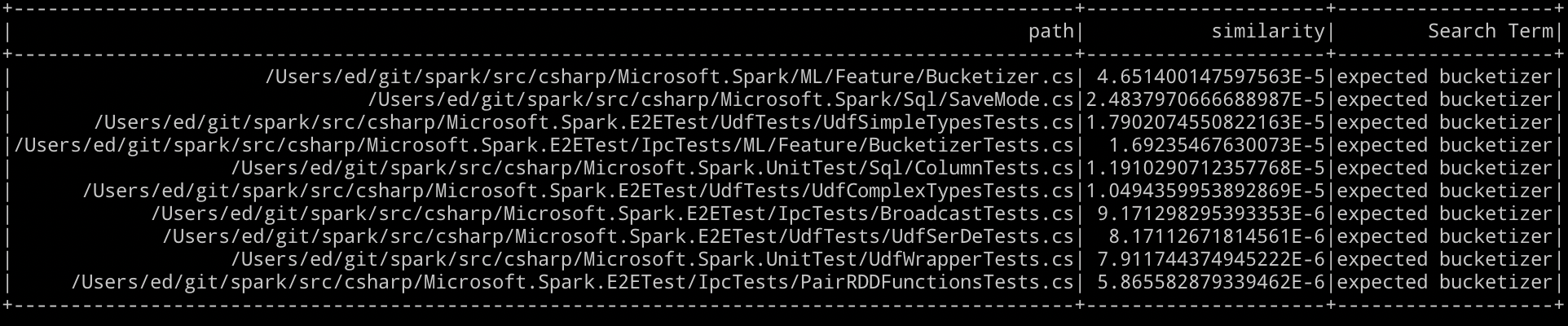### TF-IDF in .NET for Apache Spark Using Spark ML

Last Updated: 2020-10-18

NOTE: What you read here was before .NET for Apache Spark 1.0 which includes everything we need to do this purely in .NET - in this post you will see an example that is no longer necessary for TF-IDF, instead view: https://the.agilesql.club/2020/12/spark-dotnet-tf-idf.

# Spark ML in .NET for Apache Spark

Spark is awesome, .NET is awesome, machine learning (ML) is awesome, so what could be better than using .NET to write ML code in Spark?

This post is about using Spark ML API in .NET, for more info on the .NET for Apache Spark project see: https://github.com/dotnet/spark

## What can you do in Spark for Machine Learning?

Native spark has two API's for creating your ML applications. The historical one is Spark.MLLib and the newer API is Spark.ML. A little bit like how there was the old RDD API which the DataFrame API superseded, Spark.ML supersedes Spark.MLLib.

At the end of last year, .NET for Apache Spark had no support for either the Spark.ML or Spark.MLLib API's so I started implementing Spark.ML. In a similar way that .NET for Apache Spark supports the DataFrame API and not the RDD API, I started with Spark.ML and I believe that having the full Spark ML API will be enough for anyone.

The Spark ML API is broadly broken up into:

• Basic statistics
• Data sources
• Extracting, transforming and selecting features
• Classification and Regression
• Clustering
• Collaborative filtering
• Frequent pattern mining
• Model selection and tuning

## Where are we now?

Well, a few months on and a few of the objects have been implemented, there is a long way to go to complete the Spark ML API, but it is a start (Note, Covid-19 has dramatically decreased my appetite for extra work so that is at least one excuse for lack of progress).

The link above (https://spark.apache.org/docs/2.4.6/ml-guide.html) is a great place to start with ML in Spark. There are lots of examples of how to make useful things.

## Implemented objects

Microsoft.Spark.ML.Feature:

• Bucketizer
• HashingTF
• IDF
• IDFModel
• Tokenizer
• Word2Vec
• Word2VecModel
• CountVectorizer
• CountVectorizerModel
• FeatureHasher

If we go back to that article I mentioned earlier and the features section: https://spark.apache.org/docs/2.4.6/ml-features.html what we start with is the TF-IDF sample. TF-IDF (see https://en.wikipedia.org/wiki/Tf%E2%80%93idf) is a way to find relevant documents in a set of documents. First the technical description: " Term frequency-inverse document frequency (TF-IDF) is a feature vectorization method widely used in text mining to reflect the importance of a term to a document in the corpus” which roughly translates to “Find documents that include this term but only if the term appears lots of times in the document relative to the size of all the documents” so if we had these two documents:

“My favourite thing to do is to go for a stroll. The key to a good stroll in the park is to be able to walk in peace.”

“When I was little I used to like to run in the park, the most important thing about running in the park is that it really invigorates you and makes you feel alive. When I haven't been able to run I try to at least go for a stroll, yes a good stroll but nothing beats a good run.”

The first document is all about going for a stroll, that is the key concept. The second document mentions going for a stroll, but the fundamental concept is running. The idea of TF-IDF is that even if both documents mention the same word twice, the importance of it in the second document is less because the second document specifies other words more times.

## So how do we implement this in Spark?

Great question, first of all, we take a set of documents, then we build a model by:

• Splitting each document into a series of words using Tokenizer
• Use HashingTF to convert the series of words into a Vector that contains a hash of the word and how many times that word appears in the document
• Create an IDF model which adjusts how important a word is within a document, so run is important in the second document but stroll less important

This creates a model which we can then use to search for documents matching our term.

## And we can do all this with just what has been implemented already?

No, that would be amazing if we could, but there is a small blocker in that currently .NET for Apache Spark doesn't support “Vector” in a UDF and because, taking the model that we have and searching it for relevancy involves using a UDF we get a little stuck. What we can do, however, is use .NET to train the model and get the data ready and then pass off to Scala or python to do the searching. At some point, we will be able to do everything in .NET so we won't need to have this handoff phase, but for now, we need to be pragmatic.

# UPDATE!

With Version 1 of .NET for Apache Spark, the ArrayList type is now supported in UDF's which means we are able to pass a raw SparseVector from the JVM back to .NET which means that we can now finish the entrire application in .NET - I will create a new post and link here to show this working all in .NET at some point

## Let's do it!

### Prerequisites

To go through this demo you will need .NET core and the .NET for Apache Spark nuget package as well as a working Apache Spark installation. I would recommend, at a minumum, you get this tutorial running: https://dotnet.microsoft.com/learn/data/spark-tutorial/intro

I have put a full copy of the demo:

https://github.com/GoEddie/Spark-Dotnet-TF-IDF

There are C# (https://github.com/GoEddie/Spark-Dotnet-TF-IDF/tree/master/tf-idf/sparky) and F# (https://github.com/GoEddie/Spark-Dotnet-TF-IDF/tree/master/tf-idf/Fpark) versions of the .NET portion of the code.

We are going to walk through an example of reading a load of source code files, converting the documents, train a model on the documents and then querying the model to find out the most relevant documents. I am going to use this repo as my data source (because why not!):

https://github.com/dotnet/spark

### 2. Add some cool using references

``````using Microsoft.Spark.ML.Feature;
using Microsoft.Spark.Sql;
``````

### 3. Handle some args

We are going to want to pass in an argument for the source folder which we will search for documents and also an intermediate temp directory we will use to pass data between .NET and Scala.

``````
namespace sparky
{
internal class Program
{
private static void Main(string[] args)
{
if (args.Length != 2)
{
Console.WriteLine("Args!");
return;
}

var sourceDir = args;
var tempDir = args;

Console.WriteLine(\$"sourceDir: '{sourceDir}', tempDir: '{tempDir}'");

if (Directory.Exists(tempDir))
Directory.Delete(tempDir, true);

Directory.CreateDirectory(tempDir);
``````

### 4. Create a spark session

``````var spark = SparkSession
.Builder()
.GetOrCreate();
``````

### 5. Create an object to store documents

``````private class Document
{
public string Content;
public string Path;
}
``````

### 6. Create a method to gather our documents

``````private static List<Document> GetSourceFiles(string path)
{
var documents = new List<Document>();

foreach (var file in new DirectoryInfo(path).EnumerateFiles("*.cs",
SearchOption.AllDirectories))
{
Path = file.FullName,
});

return documents;
}
``````

### 7. Get the documents and convert to json

``````var documents = GetSourceFiles(sourceDir);
var json = JsonConvert.SerializeObject(documents);
``````

### 8. Create a JSON document that we can read into Apache Spark

``````var allDocumentsPath = Path.Join(tempDir, "code.json");
File.WriteAllText(allDocumentsPath, json);

``````

### 9. Now the interesting bit, split the documents into words

``````var tokenizer = new Tokenizer();
var words = tokenizer
.SetInputCol("Content")
.SetOutputCol("words")
.Transform(sourceDocuments);
``````

(congratulations, you just called your first Apache Spark ML function in .NET)

This pattern of create an object (Tokenizer) then call SetInputCol and SetOutputCol are used across the Spark.ML objects so get used to it. Also, note that a call to a method like SetInputCol returns a new object so you can't do something like:

``````tokenizer.SetInputCol(str)
tokenizer.SetOuputCol(str)
``````

If you do this, then you won't be changing your original tokenizer.

`words` in this context is a DataFrame that contains a column called “words” which contains each document as an array of words:### 10. Convert words into vectors

Computers are awesome, but they don't understand words very well, a hippopotamus is a massive animal but all a computer understands is that is there is a memory address with a series of bytes, it doesn't even know the difference between a pair of pants (English) and a pair of pants (American). Computers are however very good with numbers so we will use a `HasingTF` to convert our array of individual words into a set of numbers:

``````var hashingTF = new HashingTF()
.SetInputCol("words")
.SetOutputCol("rawFeatures")
.SetNumFeatures(100000);

var featurizedData = hashingTF.Transform(words);
``````The vector contains the number of words we have in each row (yellow), each word hashed into a number (green) and the number of times each word was found (red).

### 11. Fit our data to an IDF model

The IDF model takes our terms and works out for each document what the relevance of each term is in relation to the whole document, to do this we “Fit” the features, which means to train the features to create our model i.e. we take the words and documents and entire text across all of the documents and create a model that includes the term frequencies and the weight of each term relevent to the document.

``````var idf = new IDF().SetInputCol("rawFeatures").SetOutputCol("features");
var idfModel = idf.Fit(featurizedData);
var filtered = rescaled.Select("Path", "features");
``````

This means we now have the terms (yellow), the frequency of each term (green) and now the weighted importance of the term relative to the size of the document weighted by how often the word appears in all the documents(red):This is our model, and unfortunately, the end of the line for the .NET part, what we need to do is save everything and pass it over to Scala to finish the work. This might not be as crazy as it seems, you probably would train your model, deploy it and use that model later on to search against.

### 12. Save our work

``````tokenizer.Save(Path.Join(tempDir, "temp.tokenizer"));
hashingTF.Save(Path.Join(tempDir, "temp.hashingTF"));
filtered.Write().Mode("overwrite").Parquet(Path.Join(tempDir, "temp.parquet"));
idfModel.Save(Path.Join(tempDir, "temp.idfModel"));
``````

### 13. Hand over to Scala

In Scala we will load up the existing model but also pass in the term we are going to search for:

``````def main(args: Array[String]): Unit = {

val spark = SparkSession
.builder()
.config("spark.master", "local")
.getOrCreate();

import spark.implicits._

val intermediateDir = args(0)

val searchTermString = args.drop(1).mkString(" ")

``````

Later, we will calculate the cosine similarity of each document and of our search terms, to calculate this we will need to normalize each vector. What we do is multiple each item in the vectors attributes and then take the square root:

``````   def calcNorm(vectorA: SparseVector): Double = {
var norm = 0.0
for (i <-  vectorA.indices){ norm += vectorA(i)*vectorA(i) }
(math.sqrt(norm))
}
``````

We will do this for both our model and the search term.

``````  val calcNormDF = udf[Double,SparseVector](calcNorm)

val normalizedModelData = modelData.withColumn("norm",calcNormDF(col("features")))
``````

Now we move onto the actual search terms, create a data frame with the search terms:

``````val searchTerm = Seq(("1", searchTermString)).toDF("_id", "Content")
``````

We then do what we did in C# for each document, tokenizer and transform the search terms into features and then use the idfModel we created in C# to convert each word (feature) into a vector we can use to compare to each document:

``````val words = tokenizer.transform(searchTerm)
val feature = hashingTF.transform(words)

val search = idfModel
.transform(feature)
.withColumnRenamed("features", "features2")
.withColumn("norm2", calcNormDF(col("features2")))
``````

Now we have our original documents and our search term in a similar format, they both have a vector and the normalized vector, if we join them together, we can use spark to calculate the cosine similarity:

``````val results = search.crossJoin(normalizedModelData)
``````
``````    def cosineSimilarity(vectorA: SparseVector, vectorB:SparseVector,normASqrt:Double,normBSqrt:Double) :(Double) = {
var dotProduct = 0.0
for (i <-  vectorA.indices){ dotProduct += vectorA(i) * vectorB(i) }
val div = (normASqrt * normBSqrt)
if( div == 0 ) (0)
else (dotProduct / div)
}

``````

The cosine similarity, which we get by multiplying each item in the vector one by one, then dividing that value by the normalized value we already worked out.

So in Pseudo code:

``````For each item in the vector:
Multiply each value from each document against each value in our search term
Divided by
The result of multiplying the square root of each of the vectors total of each length and direction
``````

This then gives us a way to order the results by closest match first, if we run this and search for “expected bucketizer” we should see something similar to:Which looks like a pretty good match to me, “Bucketizer.cs” is relevant for bucketizer and expected is in “SaveMode.cs” a lot considering it is a small document, “UdfSimpleTypesTests.cs” has expected 12 times. Still, it is a much bigger document, so I am going to say that the term frequency compared to the inverse document frequency (TF-IDF) works pretty well using .NET for Apache Spark and a bit of Scala.

I hope you have found this useful. The full repo is available:

https://github.com/GoEddie/Spark-Dotnet-TF-IDF

When Vector makes its way into the .NET for Apache Spark repo, I will update this with a pure .NET version.